# Generalized Cross Boards

A Generalized Cross Board consists of a 3x3 center square of holes with four "arms" attached to it of length n1, n2, n3 and n4 and width three (see figure 1). The arm length can be any non-negative integer (i.e. zero arm length is allowed).Figure 1: The Generalized Cross Board

We shall denote a particular cross board by the four-tuple (n1, n2, n3, n4). Note that the standard English board is the special case (2,2,2,2), and Wiegleb's board is the special case (3,3,3,3) (ref). These two boards have square symmetry, but the generic generalized cross board has no symmetries at all.

We consider the standard peg solitaire problem, where the legal moves are those where a man is jumped over another to an empty hole, the man jumped over being removed from the board. As usual, no diagonal jumps are allowed.

We shall use Cartesian coordinates to identify all board locations, with (0,0) always the center of the cross (the unoccupied hole in Figure 1). A move shall be represented with the starting coordinate of the move followed by a direction U,D,L,R for up, down, left and right. For example the 4 moves available in Figure 1 are (2,0)L, (0,2)D, (-2,0)R and (0,-2)U. In the case of multiple jumps we can use the compact notation (0,0)RU for the two moves (0,0)R, (2,0)U. This notation is much bulkier than the standard notation for the English board, but we can use it on any board without having to redo the notation, and it is also very easy to remember.

Any generalized cross board can easily be shown to be a null-class board, meaning that any position and its complement are in the same fundamental class (ref). We consider the standard single vacancy complement problem (SVCP), where we attempt to play from a full board minus a single man to the complementary position where only the initially vacant hole contains a single man (Figure 1 shows the starting position for the (0,0) complement problem). Only on a null-class board is it possible to solve the single vacancy complement problem, but even on such a board it may not be possible to solve the SVCP at every location.

Note that if n1= n3=0, or n2= n4=0, then the generalized cross board degenerates into a rectangular board. The SVCP is always unsolvable on such boards along the center column or row. We do not consider these boards to be generalized cross boards, and we require that one of n1 or n3, and n2 or n4, be greater than zero.

We could generalize farther and allow the side of the center square and width of the arms of the cross to be larger than three. However these boards are generally not null-class boards. For this reason, we don't generalize farther.

A block removal in peg solitaire is a set of moves that removes an entire block of men. A block removal generally requires a catalyst that is restored to its original position at the completion of the moves. The most useful block removal for generalized cross boards is the six-removal of Figure 2.Figure 2: The Six-Removal

For this six-removal, the required catalyst is an unlike-pair, represented by the two U's in Figure 2. The reader can verify that if either hole is empty and the other contains a man, then there is a sequence of six moves that removes only the rightmost six men from the board and restores the two catalyst locations to their original positions.

# Solvability Extension Theorems

One fundamental property of generalized cross boards is that if the SVCP is solvable at some location (x,y) on a board (n1, n2, n3, n4), then usually the same location is solvable on all larger boards where each arm is increased in length by any even amount. It is not hard to see why this is the case. Suppose we take a solution to the SVCP at (x,y). As this solution is played, it is likely that at some point the tip of the arm n1 contains an unlike pair on the outside of the tip as in Figure 3.Figure 3

Where the locations marked by U or V denote an unlike-pair (note that if n1<2 the unlike pair may lie inside the center portion of the cross). At this point in the solution, one can insert a six-removal to produce a solution to the SVCP at (x,y) for the board (n1+2, n2, n3, n4). In fact, one may extend n1 by any even amount i1 by introducing i1/2 six-removals to create a solution to the SVCP at (x,y) on the board (n1+i1, n2, n3, n4). To recursively apply multiple six-removals, one executes the first one until an unlike pair appears in the proper position relative to the next one. This then becomes the catalyst for the next six-removal and the first one is finished after the second is completed.

A solution to the SVCP at (x,y) is called arm-clearing if, for each of the four arms, at some point during the solution the tip of that arm shows an unlike pair on either side (as in Figure 3). By inserting 6-removals at these points in the solution, we can produce a solution to any larger board where each arm is increased in length by any even amount (or zero). We can restate this as:

Extension Theorem 1: If an arm-clearing solution to the SVCP at (x,y) exists for the board (n1, n2, n3, n4) then the same location (x,y) is solvable on any board (n1+ i1, n2+ i2, n3+ i3, n4+ i4) for any non-negative even integers i1, i2, i3, i4.

If a board location is solvable, does an arm-clearing solution always exist? The answer is no, as we shall show. However, in the majority of cases, an arm-clearing solution does exist. It is not hard to search for arm-clearing solutions using a computer.

Because of Extension Theorem 1, all generalized cross boards are divided into 6 categories depending on the evenness or oddness of their arm lengths. Abbreviating E for even and O for odd, we can denote these categories depending on the parity of the arm lengths as we move around the cross: OOOO, OEEE, OOEE, OEOE, EOOO, and EEEE. To determine the solvability of location (x,y) on a board, we can look for arm-clearing solutions at (x,y) on all smaller boards in the same category.

Extension Theorem 2: If the board (n1, n2, n3, n4) is solvable at A (Figure 4), then the board (n1+2, n2, n3, n4) is solvable at A'. Similarly for B and B', and C and C' (both C' locations).Figure 4

The proof of this is simple: one can show that a solution to the larger board at A' can be constructed from the solution to the smaller board at A by inserting six additional moves. We insert two moves at the beginning to reproduce the SVCP at A on the smaller board, and four moves at the end to finish the solution off. The reader may wish to verify these simple extension moves.

Both extension theorems are useful only for solvable board locations. If a certain board location is not solvable, then nothing at all can be inferred about its solvability on a larger board. Also note that we can not conclude anything about the solvability in the middle of the tip of any arm that has increased in length (the two locations marked by a ? in Figure 4). We shall see that in some sense these are the hardest locations to solve the SVCP.

# Solvable Boards

We now ask the basic question: which generalized cross boards have the SVCP solvable everywhere?? We shall call such boards solvable boards. At this point we know only that the standard English board (2,2,2,2) is solvable (ref).

First, we consider boards whose arm lengths ni are all less than or equal to 3. There are 45 unique boards satisfying this constraint. Using a computer, we can easily classify all board locations as solvable or unsolvable. In addition, for the solvable locations, we can try to find arm-clearing solutions. The best strategy is to consider each category of board separately, and solve all the boards in this category from smallest to largest. By using the extension theorems, only a few locations on the larger boards need to be checked by the computer for solvability.

Often the solvability of a board may be completely determined by smaller boards using the extension theorems. For example, in the category EOOO, the board (3,3,2,1) is solvable, and moreover at all locations an arm-clearing solution can be found. The board (3,3,3,2) is an extension of the smaller board, and moreover it can be considered an extension in two different ways (either by rotating counter-clockwise by 90 degrees or by flipping it around the x-axis and rotating counter-clockwise by 90 degrees). These two extensions cover all of the (3,3,3,2) board, hence it too must be solvable and every location has an arm-clearing solution.

Tables 1.1-1.6 show all 45 such boards by category, with the solvability of all locations identified. With the single exception noted [on the OEOE (3,2,1,2) board], all solvable locations have an arm-clearing solution. Note that if the solvable locations are listed in the comments, one can assume that all other locations are unsolvable (and vice-versa).Table 1.1: OOOO BoardsTable 1.2: EOOO Boards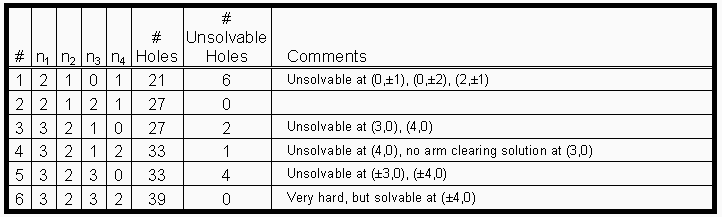Table 1.3: OEOE Boards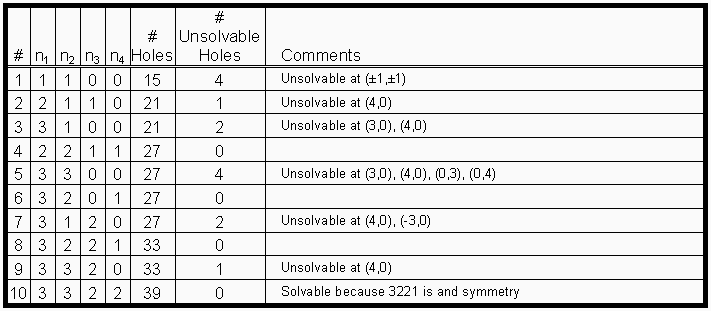Table 1.4: OOEE Boards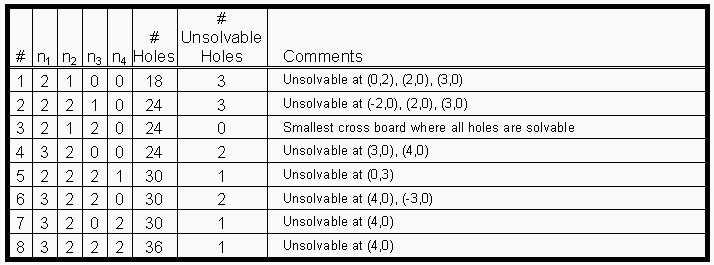Table 1.5: OEEE Boards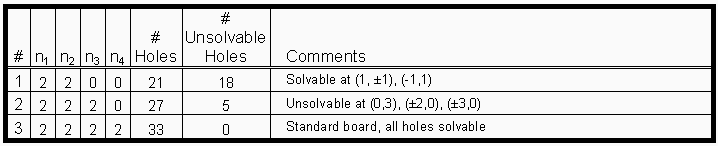Table 1.6: EEEE Boards

# Larger Generalized Cross Boards

What about the solvability of boards with arms longer than 3? Using the results of the last section, coupled with the extension theorems, it is quite easy to show that all sufficiently large generalized cross boards are solvable at all nine center holes. By "nine center holes" we mean {(x,y) such that |x|<2, |y|<2}. In particular, if a board is of category C, and has at least n arms of length two or greater, then the SVCP is solvable at all nine center holes. This is most easily accomplished on the boards of category C=OEEE or OOEE, which only require n=1, while for C=OEOE or EOOO we must require n=2 and for C=OOOO or EEEE we must require n=3.

However, solvability of the entire board requires solvability on all arms as well. In particular the middle of the tip of the longest arm must be solvable. Solving any board here is tricky because we must essentially clear this arm out and then at the end march back into this arm to leave the last man at the tip. In a general sense it is related to Conway's problem of sending a man out five holes (ref). This problem uses an infinite board (no boundaries), and begins with an arbitrary number of men behind the line x=0. The goal is to send a man out in x as far as possible. Conway's result is that it is impossible to send a man out 5 (or more) spaces.

Using the computer, one can search for boards with arms of length 4 that are solvable at the middle of the tip of the longest arm. However, searching over increasingly large boards becomes very time consuming. It turns out one can prove that such a search is fruitless.

Theorem: No generalized cross board with an arm longer than 3 is solvable.

The proof of this is, unfortunately, much more tedious than the elegant proof of Conway's result. The proof consists of three steps:

Step 1) Prove that a board with all ni<=4, and at least one arm of length 4 cannot be solvable.

Step 2) Prove that a board with an arm of length 5 or greater cannot be solvable.

Step 1 outline:
There are 60 unique boards that fall into this category. While some of these boards can be proven unsolvable by using carefully chosen resource counts, a lot of them can not. We can try to solve a more general problem by allowing any integer number of pegs (positive or negative) at each board location. A "move" in this case is an operation on 3 consecutive cells, where we add -1 to two consecutive cells and +1 to the third. Looked at it this way the peg solitaire problem is an integer programming model, and Beasley calls this "Unconstrained Solitaire".

If the original peg solitaire problem is solvable, then the unconstrained solitaire problem must also be solvable. Therefore if we can prove that the unconstrained problem is unsolvable, it will prove that the original peg solitaire problem is unsolvable as well. However, if we apply this technique to the boards of Step 1, we find that most of them are solvable in unconstrained solitaire.

The trick here is to add exit constraints directly to the integer programming model. These are extra constraints that any solution to the peg solitaire problem must satisfy. If we consider a region R that begins full but ends empty, we call a move an exit from this region if it removes one or more pegs from the region and places a peg outside it. If the region R has at least three holes, the exit theorem states that there must be at least two exits from this region (see Beasley's book for a complete statement and examples of the exit theorem).

Consider the 3 hole region {(2,1),(3,1),(4,1)}. By the above theorem, this region must have at least 2 exits, and in this case an exit must be a move that starts in the region but ends outside it. There are six such moves {(2,1)L, (3,1)L, (3,1)R, (2,1)D, (3,1)D, (4,1)D} (recall that this arm has length 4, so the move (4,1)R is not possible) and we can add these constraints directly to our integer model. We repeat this for the other 7 such regions symmetrically about the board (2 regions in each arm), as long as the arm has length at least 3. For the arms of length 2 or less, we don't add any exit constraints.

Now when we feed this model into an integer programming solver, it comes back with "integer infeasible" for all 60 of the possible boards. This is a proof that the single vacancy complement problem on these boards is not solvable at the center tip of the longest arm. It is somewhat unsatisfying, however, as the IP model does not give any intuition as to why the model is infeasible. Also, it's always possible that due to some programming error this result is actually incorrect.

The results appars to be valid, however. I have also applied this integer model to Wiegleb's board (3,3,3,3) which also comes out unsolvable at (4,0). Thus the technique agrees with previously known results (Beasley, p. 201). The integer programming model does not come back integer infeasible for the board (3,2,3,2), and any that we know to be solvable.

Step 2 outline:
Here we use the resource count using the golden ratio used by Conway. If the initial vacancy is at the tip of the right arm (the board location (6,0) in Figure 1), then there are essentially only two possible ways to clear this arm and deliver the final peg to (6,0). After the first move, there will be a peg at (6,0) and this can be cleared by either a horizontal jump (6,0)L or a vertical jump (6,-1)U or (6,1)D. In the first case all jumps in the arm must be horizontal jumps. The jumps needed to clear the arms incur a debt at the board locations at the base of the arm. The longer the arm is, the greater this debt.

It can be shown that the debt incurred is too great to erase if the arm to be cleared is long enough or the rest of the board is small enough. The only case in step 2 which cannot be proven by the resource count is the board (5,5,5,5), which can easily be treated as a separate case by the integer programming technique of step 1. All other boards in step 2 can be shown unsolvable by the resource count.

# Summary

We have shown that there are exactly 12 generalized cross boards where the single vacancy complement problem is solvable at every board location. The only board of the 12 with square symmetry is the standard English Board (2,2,2,2). There are two boards which have rectangular symmetry around both x and y axes:Figure 5: The 27 hole board (2,1,2,1) and 39 hole board (3,2,3,2)

There are three boards which have rectangular symmetry about only one axis: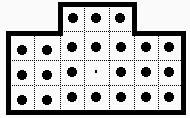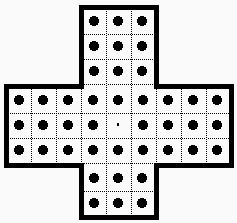Figure 6: The 24 hole board (2,1,2,0), 36 hole board(3,2,3,1), and 42 hole board (3,3,3,2)

Note that the (2,1,2,0) board is the smallest solvable generalized cross board, and (3,3,3,2) is the largest (in terms of number of holes).

There are two boards with diagonal symmetry about the line X=Y:Figure 7: The 27 hole board (2,2,1,1) and 39 hole board(3,3,2,2)

And finally, the 4 remaining solvable boards have no symmetries at all: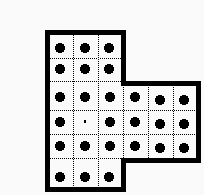Figure 8: The 27 hole board (3,2,0,1), 30 hole board(3,2,1,1)
33 hole board (3,2,2,1) and 36 hole board (3,3,2,1)

Three of these new boards can be played on a standard (English) solitaire board by masking off some of the holes. The reader may enjoy attempting some of the single vacancy complement problems on these boards. An actual Wiegleb's solitaire board is not easy to find, but if you have one you can play all 12 solvable boards by masking off certain holes.Peg Solitaire Main Page ...